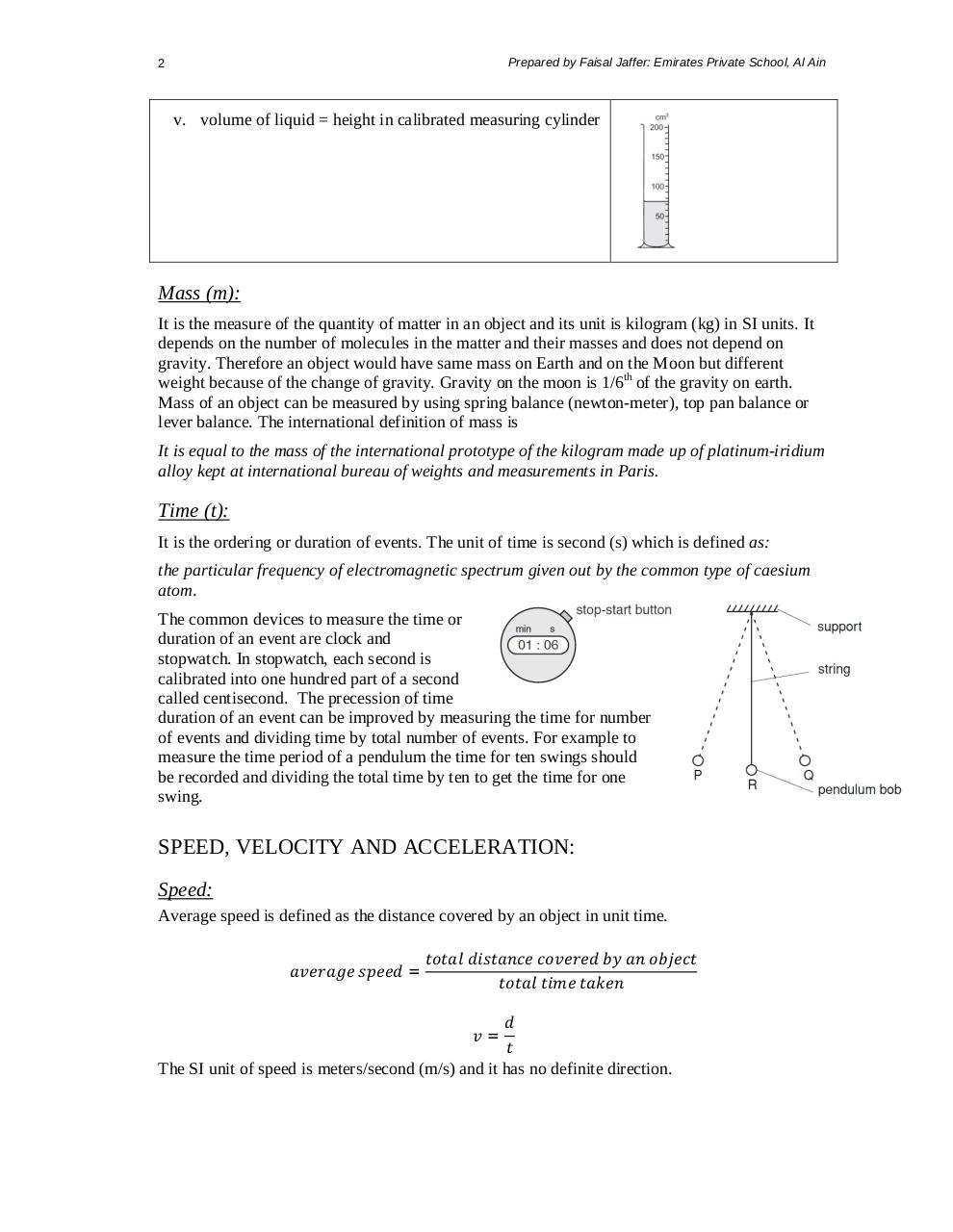# Summary of Chapter 2,3,4.pdfPage 1 23417

#### Text preview

Prepared by Faisal Jaffer: Emirates Private School, Al Ain

2

v. volume of liquid = height in calibrated measuring cylinder

Mass (m):
It is the measure of the quantity of matter in an object and its unit is kilogram (kg) in SI units. It
depends on the number of molecules in the matter and their masses and does not depend on
gravity. Therefore an object would have same mass on Earth and on the Moon but different
weight because of the change of gravity. Gravity on the moon is 1/6th of the gravity on earth.
Mass of an object can be measured by using spring balance (newton-meter), top pan balance or
lever balance. The international definition of mass is
It is equal to the mass of the international prototype of the kilogram made up of platinum-iridium
alloy kept at international bureau of weights and measurements in Paris.

Time (t):
It is the ordering or duration of events. The unit of time is second (s) which is defined as:
the particular frequency of electromagnetic spectrum given out by the common type of caesium
atom.
The common devices to measure the time or
duration of an event are clock and
stopwatch. In stopwatch, each second is
calibrated into one hundred part of a second
called centisecond. The precession of time
duration of an event can be improved by measuring the time for number
of events and dividing time by total number of events. For example to
measure the time period of a pendulum the time for ten swings should
be recorded and dividing the total time by ten to get the time for one
swing.

SPEED, VELOCITY AND ACCELERATION:
Speed:
Average speed is defined as the distance covered by an object in unit time.
=

=
The SI unit of speed is meters/second (m/s) and it has no definite direction.